# AP Board 3rd Class Maths Solutions 5th Lesson Multiplication

## AP State Syllabus 3rd Class Maths Solutions Chapter 3 Addition

Textbook Page No. 58

I.
In 16 × 3 = 48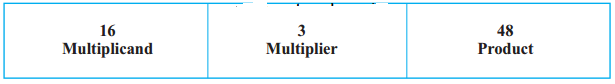In 21 × 2 = 42,
Which one is multiplicand ?
21

Which one is multiplier ?
2

Which one is product ?
42

Write multiplicand, multiplier and product in the following multiplications :

1. In, 8 × 2 = 16
Multiplicand = _____
Multiplier = ____
Product = ____

2. In, 30 × 3 = 90
Multiplicand = ____
Multiplier = ____
Product = ____3. In, 91 × 1 = 91
Multiplicand = _____
Multiplier = ____
Product = ____

Textbook Page No. 59

Do these:

1. Do the following

a)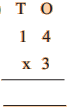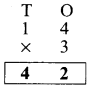b)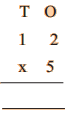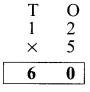c)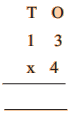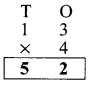Question 2.
Karthik had 4 packets. In each packet, there are 24 crackers. How many crackers are there in all?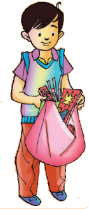Crackers in each packet = 24
Number of packets = ____
Total number of crackers
= 24 × ____ = ______
Crackers in each packet = 24
Number of packets = 4
Total number of crackers
= 24 × 4 = 96

Textbook Page No. 60

Question 3.
There are 12 bananas in a bag. Find the number of bananas in 5 such bags.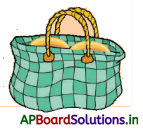Number of bananas in each bag = _____
Number of bags = 5
Total number of bananas = ____ × 5 = _____
= ______
Number of bananas in each bag = 12
Number of bags = 5
Total number of bananas = 12 × 5 = 60

Do these :

1. Do the following.

a) 86 × 2 = ____
172

b) 64 × 3 = ____
192

c) 45 × 5 = ____
225

d) 58 × 4 = ____
232

Question 2.
There are 50 books in a box and there are 4 such boxes. Find the total number of books.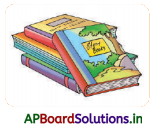Number of boxes =
Number of books in each box =
Total number of books =
Number of boxes = 50
Number of books in each box = 4
Total number of books = 50 × 4 = 200Question 3.
Cost of a pen is ₹ 4. What is the cost of 32 pens ?
Cost of a pen is = ₹ 4
Cost of 32 pens = 32 × 4 = ₹ 128

Question 4.
There are 63 sweets in a box. How many sweets are there in 3 such boxes ?
Number of sweets in a box = 63
Number of such boxes = 3
Total sweets in 3 boxes = 63 × 3
= 189 sweets

Question 5.
52 people can travel in a bus. How many people can travel in 4 such buses ?
Capacity of a bus = 52 people
Number of buses = 4
Total people can travel in 4 buses = 52 × 4 = 208 people

Textbook Page No. 63

Do these

Question 1.
Make table 7 by using Table 5 and Table 2.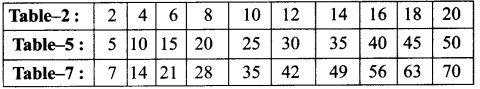Question 2.
Make table 9 by using Table 7 and Table 2.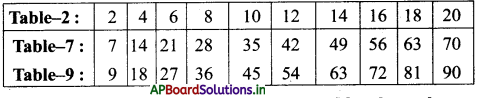Question 3.
Make table 8 by using any two relevant tables chosen by you.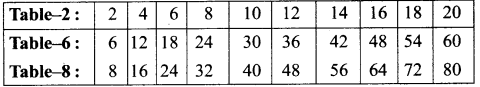Textbook Page No. 65

Exercise – 1

1. Write multiplicand, multiplier and product in the following multiplications:

a) 72 × 4 = 288;
Multiplier = ____;
Multiplicand = ____;
Product = ____b) 5 × 100 = 500;
Multiplier = ____;
Multiplicand = ____
Product = _____
500

c) 84 × 1 = 84;
A. Multiplier = _____;
Multiplicand = ____;
Product = ___

d) 24 × 24 = 576;
Multiplier = ____;
Multiplicand = _____
Product = _____;

2. Multiply the following.

A. a) 75 × 2 = ___
150

b) 95 × 4 = ____
380

c) 70 × 8 = ____
560

d) 93 × 9 = ____
837

e) 64 × 8 = ____
512

f) 96 × 10 = ____
960

g) 20 × 10 = ____
200

h) 75 × 10 = ____
750

i) 55 × 10 = ___
550

c) Prepare table for 9 using the table 5 and 4

3.
a) Prepare table for 5 using the tables 2 and 3.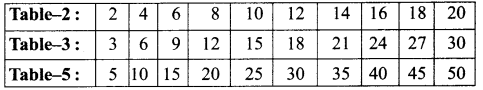b) Prepare table for 10 using the table 6 and 4.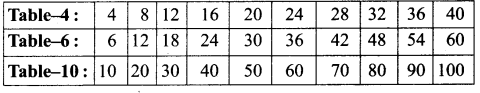c) Prepare table for 9 using the table 5 and 4.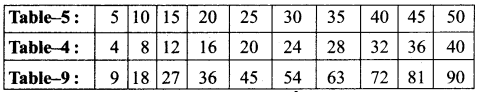4. Work out the following.

a) The cost of one pencil is ₹ 6. What is the cost of 72 such pencils ?
Cost of one pencil = ₹ 6
Cost of 72 pencils = 72 × 6
Total amount = ₹ 432b) In an orchard, there are 10 rows of mango of trees and each row contain 25 trees. How many mango trees are there in total ?
Number of rows in orchard = 10
Number of trees in each row = 25
Total trees in the orchard
= 25 × 10 = 250

Textbook Page No. 68

1. Do the following.

a)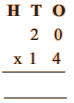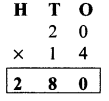b)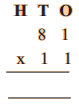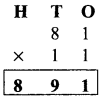c)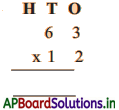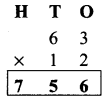d)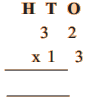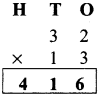Question 2.
There are 24 hours in a day. How many hours are there in 11 days ?
Number of hours in a day = 24
Number of days = 11
Number of hours in 11 days
= 11 × 24 = 264hrs

Question 3.
Cost of Black gram per Kg is ₹ 90, how much money has to be paid for 13 Kg ?
Cost of Black gram per Kg = ₹ 90
Number of Kgs = 13
Total money paid = 13 × 90 = ₹ 1170

Textbook Page No. 70

1. Do the following.

a)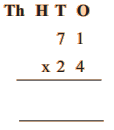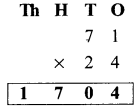b)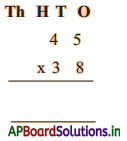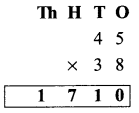c)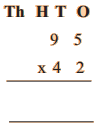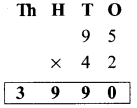Question 2.
48 people can travel in a bus. How many people can travel in 26 such buses ?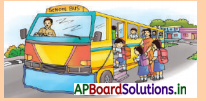Number of people can travel in a bus = 48 people
Number of buses = 26 buses
Number of people can travels in 26 buses = 26 × 48 = 1248Question 3.
In a library, there are 48 cupboards and in each cupboard, there are 63 books. How many books are there in the library ?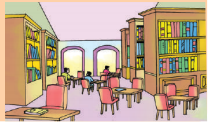Number of cupboards in a library = 48
Number of books in each
cup board = 63 books Total books in the library = 48 × 63
= 3,024 books

Textbook Page No. 71

Do these:

Fill in the blanks.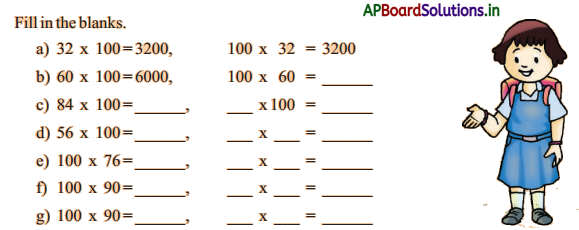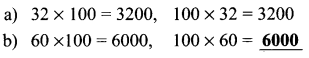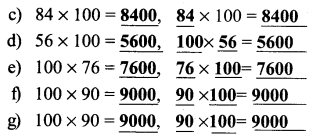Textbook Page No. 73

Do these:

1. Do the following.

a)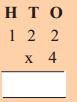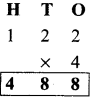b)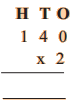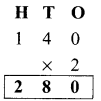c)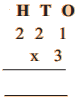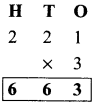d)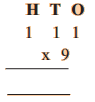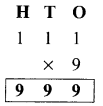Question 2.
A book contains 130 pages. Total how many pages are there in 3 such books ?
Number of pages in a book = 130 pages
Number of books = 3
Total pages = 3 × 130 = 390 pages

Question 3.
A bag costs ₹ 300. How much money will be paid to buy 2 bags ?
Cost of each bag = ₹ 300
Number of bags = 2
Cost 2 bags = 2 × 300 = ₹ 600

Question 4.
There are 2 boxes and in each box there are 142 balls. How many balls are there in all ?
Number of balls in each box = 142
Number of boxes = 2
Total balls in 2 boxes = 2 × 142
= 284 balls

Exercise – 2

1. Multiply:

a) 24 × 3 = ____;
3 × 24 = ____;
24 × ___ = 3 = 3 × ___ = ____
24 × 3 = 3 × 24 = 72

b) 100 × 1 = ____;
1 × 100 = _____;
100 × ___ = 1 × ____ = ____
100 × 1 = 1 × 100 = 100

c) In 53 × 27 = 1431 ;
Multiplicand = _____
Multiplier = _____
Product = _____

d) In 321 × 3 = 963 ;
Multiplicand = _____
Multiplier = _____
Product = 963

e) In 108 × 2 = 216;
Multiplicand = _____;
Multiplier = ____;
Product = _____

2. Multiply:
a)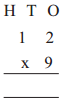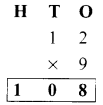b)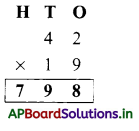c)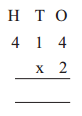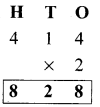3. Fill in the blanks.

a) 67 × 5 = ____
335

b) 93 × 4 = ____
372

c) 123 × 3 = ____
369

Question 4.
There are 36 beads in a necklace. How many beads are there in 13 necklaces ?
Number of necklaces = 13

Question 5.
If there are 48 bottles in one carton, how many bottles are there in 16 cartons ?
Number of bottles in a carton = 48
Number of cartons = 16
Total bottles in cartons
= 16 × 48 = 768 bottles

Question 6.
There are 54 grapes in one bunch. If, there are 44 such bunches, how many grapes are there ?
Number of grapes in a bunch = 54 grapes
Number of bunches = 44
Total grapes in 44 bunches = 44 × 54
= 2376 grapes

Question 7.
Cost of dictionary is ₹ 120. How much money he has to pay for 4 dictionaries ?
Cost of a dictionary is = 120
Number of dictionaries =4
Total money has to pay
for 4 dictionaries = 4 × 120 = ₹ 480

Question 8.
5 students of a class collected ₹ 110 each for the Prime Minister’s relief fund. How much money did they collect altogether ?
Number of students for each class = 5
Collected money for each class = ₹ 110
Total collected money = ₹ 110 × 5
= ₹ 550

Multiple Choice Questions

Question 1.
Repeated addition is called ( )
B) Subtraction
C) Multiplication
D) DivisionQuestion 2.
In the multiplication which is on the right side of the symbol ‘=‘ is called ( )
A) Multiplicand
B) Multiplier
C) Product
D) All
C) Product

Question 3.
In 21 × 2 = 42, 21 is called ( )
A) Multiplicand
B) Multiplier
C) Product
D) All
A) Multiplicand

Question 4.
In 17 × 4 = 68, 4 is called ( )
A) Multiplicand
B) Multiplier
C) Product
D) All
B) Multiplier

Question 5.
In 45 × 3 = 145, 145 is called ( )
A) Multiplicand
B) Multiplier
C) Product
D) All